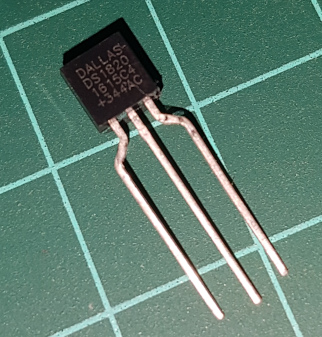# DS18S20 Temperature Sensor

December 2nd, 2021I believe there is a mistake in calculation of the fine part of the temperature value as received from 1-Wire DS18x20-family sensors in the linux driver. The current implementation does indeed follow the official datasheet, but exactly the datasheet seems to be incorrect in this place.

DS18S20 Datasheet says two different things on the Page 6:

First:

Resolutions greater than 9 bits can be calculated using the data from the temperature, COUNT REMAIN and COUNT PER °C registers in the scratchpad. Note that the COUNT PER °C register is hard-wired to 16 (10h). After reading the scratchpad, the TEMP_READ value is obtained by truncating the 0.5°C bit (bit 0) from the temperature data (see Figure 4).

And then:

"The extended resolution temperature can then be calculated using the following equation: TEMPERATURE = TEMP_READ - 0.25 + (COUNT_PER_C - COUNT_REMAIN) / COUNT_PER_C

The last one is the formula that is implemented in the linux driver. I have performed live tests with DS18S20 sensors to verify it, how they behave. I was lowering the temperature in slow steps to see individual transitions. Below is the result.

(value), 1 (sign), 6 (count remain) and 7 (count per degC)

Response bytes
0 value : 1 sign ::
6 count rem : 7 count per degC
Byte 0 binary Fraction =
Byte 7 minus Byte 6
Bits 7..1 of Byte 0
Bits 3..0 of Fraction
Hex Multiplied by 1/16°C
37:00::04:10 0011 0111 0C = 1100 0011011.1100 1BC 27.75
37:00::05:10 0011 0111 0B = 1011 0011011.1011 1BB 27.6875
37:00::06:10 0011 0111 0A = 1010 0011011.1010 1BA 27.625
37:00::07:10 0011 0111 09 = 1001 0011011.1001 1B9 27.5625
37:00::08:10 0011 0111 08 = 1000 0011011.1000 1B8 27.5
36:00::09:10 0011 0110 07 = 0111 0011011.0111 1B7 27.4375
36:00::0A:10 0011 0110 06 = 0110 0011011.0110 1B6 27.375
36:00::0B:10 0011 0110 05 = 0101 0011011.0101 1B5 27.3125
36:00::0C:10 0011 0110 04 = 0100 0011011.0100 1B4 27.25
36:00::0D:10 0011 0110 03 = 0011 0011011.0011 1B3 27.1875
36:00::0E:10 0011 0110 02 = 0010 0011011.0010 1B2 27.125
36:00::0F:10 0011 0110 01 = 0001 0011011.0001 1B1 27.0625
36:00::10:10 0011 0110 00 = 0000 0011011.0000 1B0 27
35:00::01:10 0011 0101 0F = 1111 0011010.1111 1AF 26.9375
35:00::02:10 0011 0101 0E = 1110 0011010.1110 1AE 26.875
35:00::03:10 0011 0101 0D = 1101 0011010.1101 1AD 26.8125
35:00::04:10 0011 0101 0C = 1100 0011010.1100 1AC 26.75
35:00::05:10 0011 0101 0B = 1011 0011010.1011 1AB 26.6875
35:00::06:10 0011 0101 0A = 1010 0011010.1010 1AA 26.625
... ... ... ... ... ...
00:00::0E:10 0000 0000 02 = 0010 0000000.0010 002 0.125
00:00::0F:10 0000 0000 01 = 0001 0000000.0001 001 0.0625
00:00::10:10 0000 0000 00 = 0000 0000000.0000 000 0
FF:FF::01:10 1111 1111 0F = 1111 1111111.1111 FFF -0.0625
FF:FF::02:10 1111 1111 0E = 1110 1111111.1110 FFE -0.125
... ... ... ... ... ...
FC:FF::0F:10 1111 1100 01 = 0001 1111110.0001 FC1 -1.9375
FC:FF::10:10 1111 1100 00 = 0000 1111110.0000 FC0 -2.0000
FB:FF::01:10 1111 1011 0F = 1111 1111101.1111 FBF -2.0625

My findings are confirmed by other people, e.g. here: electro-tech-online

The only unclear thing for me is the Byte 7. The datasheet says it is hard wired to 10h. The guy here says, he has seen devices with other dividers: electro-tech-online

I noticed that the count divisor was not equal to 16 as the data sheet indicated it would be. In fact, as the temperature was raised and lowered, the count divisor would increase and decrease in value (not fixed).

Assuming the guy did not make any mistake, it would be interesting to know, whether the divisor is always a power of 2 (which would make the conversion easy) or can be of any value.

Helpful in answering the question may be the datasheet of the original DS1820 sensor (without the 'S'), especially the section "OPERATION – MEASURING TEMPERATURE". The operation of the sensor can be simplified as follows: there is an RC-oscillator circuit of a frequency dependent on the temperature. The number of oscillations is counted within a time window (gate period). The "count remain" is the value that remains after the gate has been closed. As we know how many cycles do correspond to a single degree, we can calculate this fraction easily. The document says, the oscillator has a non-linear characteristics over the temperature and must be therefore compensated. This clarifies the dynamic value of the "count per degree" field. Maybe future releases of the sensor introduced a linear oscillator and fixed the value to 10h?

Next: SanDisk SecureAccess AES 1024bit

Previous: OpenTTD Germany and XL Europe Map